# Binary form

A form in two variables, viz. a homogeneous polynomialwhere the coefficients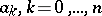belong to a given commutative ring with a unit element. Such a ring may be the ring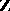of integers, the ring of integers of some algebraic number field, the fieldof real numbers or the field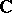of complex numbers. The numberis called the degree of the form. If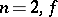is called a binary quadratic form.

The theory of forms includes algebraic (theory of invariants), arithmetic (representation of numbers by forms) and geometric (theory of arithmetical minima of forms) approaches. The purpose of the algebraic theory of binary forms (inor) is to construct a complete system of invariants of such forms under linear transformations of variables with coefficients of the same field (cf. Invariants, theory of; see also , Chapt. 5). The arithmetic theory of binary forms studies Diophantine equations of the form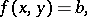where, their solvability and their solutions in the ring. The most important result is Thue's theorem and its generalizations and sharpenings (cf. Thue–Siegel–Roth theorem). See , Chapts. 9–17, and the Mordell conjecture on the solvability of such equations in the field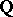and the possible number of solutions. The theory of arithmetical minima of binary forms is part of the geometry of numbers. The arithmetical minimum of a formis defined as the quantity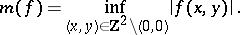It has been proved for the case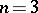that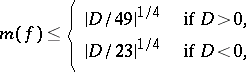whereis the discriminant of, which, in the present case, is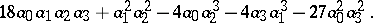These estimates cannot be improved.